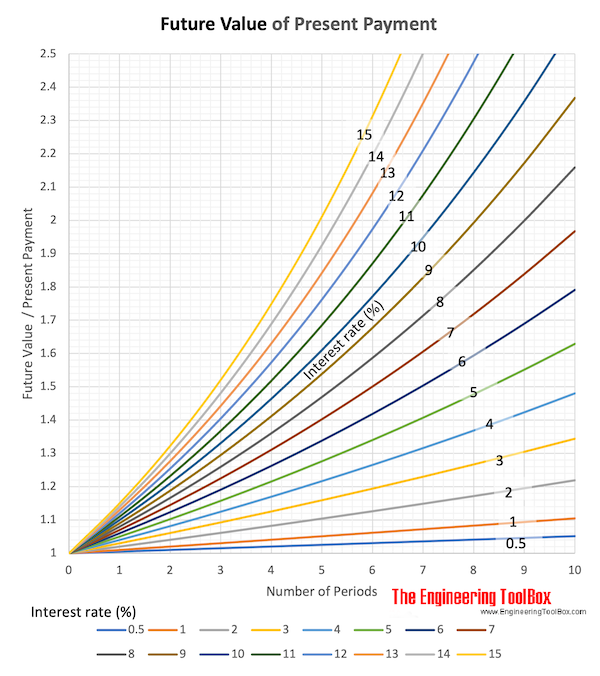Engineering ToolBox - Resources, Tools and Basic Information for Engineering and Design of Technical Applications!

# Interest Rate

## Interest rate is the cost of money.

Interest is the cost of money and is measured by the interest rate where

interest rate = cost of having money available for use

The interest rate is a percentage that is periodically added to an amount of money over a specified length of time. If money is borrowed the interest rate is the percentage of the borrowed amount that is paid to the borrower as a compensation for the use of the borrowed property.

Interest reflects that

• money available today has a greater value that money received in the future

The future value of a present amount of value can be expressed as

F = P (1 + i)n                     (1)

where

F = future value

P = present value

i = interest rate per period

n = number of interest periods

### Example - Accumulated Value

The accumulated value of an amount of present value 1 today with interest rate 10% in a 10 years period can be expressed as

Accumulated Value Year by Year with Interest Rate 10%
YearAccumulated Value
0 1
1 1.1
2 1.21
3 1.33
4 1.46
5 1.61
6 1.77
7 1.94
8 2.14
9 2.36
10 2.59

An amount of 500 will accumulate with the factor 2.14 in 8 years - or

F = 2.14 (500)

= 1070

### Future Value of Present Money due to Interest Rate - Calculator Table

This calculated table visualizes the increase in future value of present money due to interest rate and time.### Finding Unknown Interest Rate

If a single payment P shall produce a future value F after n annual compounding periods, the per annum decimal interest rate can be calculated as

i = (F / P)1/n - 1                              (2)

#### Example - Finding Interest Rate

A single payment of 100 today shall grow to 120 in 5 years. The required interest rate can be calculated as

i = (120 / 100)1/5 - 1

= 0.037

= 3.7 %

## Related Topics

• ### Economics

Engineering economics - cash flow diagrams, present value, discount rates, internal rates of return - IRR, income taxes, inflation.

## Related Documents

• ### Cash Flow Diagrams

The future value of money.
• ### Compound Interest Tables

Compound interest tables - interests rates 0.25 - 60%.
• ### Contract Types

Commonly used engineering and construction contracts.
• ### Discrete Compounding Cash Flow Formulas

Discrete payments compounding equations and online calculators .
• ### Interest Formulas

Future value of single cash flow.
• ### Internal Rate of Return - IRR

Internal Rate of Return (IRR) - the break-even interest rate.
• ### Net Present Worth (NPW) of a Cash Stream

The value of a stream of payments is called the Net Present Worth (NPW).
• ### Present Value

The value of money in the future is the Present Value.

## Engineering ToolBox - SketchUp Extension - Online 3D modeling!

Add standard and customized parametric components - like flange beams, lumbers, piping, stairs and more - to your Sketchup model with the Engineering ToolBox - SketchUp Extension - enabled for use with older versions of the amazing SketchUp Make and the newer "up to date" SketchUp Pro . Add the Engineering ToolBox extension to your SketchUp Make/Pro from the Extension Warehouse !

We don't collect information from our users. More about

## Citation

• The Engineering ToolBox (2008). Interest Rate. [online] Available at: https://www.engineeringtoolbox.com/interest-rate-d_1229.html [Accessed Day Month Year].

Modify the access date according your visit.

9.27.11